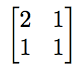On a recent episode of our podcast My Favorite Theorem, my cohost Kevin Knudson and I talked with Carina Curto, a mathematician at Penn State University who specializes on mathematics applied to biology and neuroscience. You can listen to the episode here or at kpknudson.com, where there is also a transcript.

Curto told us about the Perron-Frobenius theorem, which comes from the field of linear algebra. As Curto gushes in the episode, linear algebra is one of the workhorses of mathematics. It provides a robust set of techniques for modeling and understanding systems of equations that arise in many different contexts.

One of the central objects of study in linear algebra is a matrix. A matrix is an array of numbers, but it’s much more than that. The numbers are arranged in rows and columns and are usually thought of as a shorthand representation for a transformation of a space. For example, one way to transform the plane is to take each point in the plane, written as (x,y) and send the first coordinate, x, to the sum 2x+y and the second coordinate, y, to x+y. The matrixencodes that transformation.

In general, a ray of points emanating from the origin can be stretched and rotated by a transformation encoded by a matrix, but in a special direction called the eigenvector, the transformation is limited to stretching or shrinking. There is no rotation. The amount by which a unit length segment pointing in this direction is stretched or shrunk is the eigenvalue. The Perron-Frobenius theorem states that for a square matrix with all positive entries, there is a unique largest real eigenvalue and that its corresponding eigenvector has positive x and y coordinates.

I vaguely remembered hearing about the Perron-Frobenius theorem before talking with Curto, but I did not initially find it particularly compelling. I learned early in my first linear algebra class not to put too much stock into the numbers you see in a matrix. That is, you shouldn’t think you can understand what a matrix will do based only on what its entries look like. I think part of my failure to appreciate the Perron-Frobenius theorem earlier is that I was a little bit suspicious of a theorem that did allow me to know something about a transformation based on the numbers in a matrix. And to top it off, not many matrices have all positive entries, so what’s the point of a theorem that applies to such an exclusive set of matrices?

Curto convinced me that the theorem is more useful than I gave it credit for. True, there’s no reason to think any arbitrary matrix is going to have all-positive entries, but many applications do deal primarily with all-positive matrices. The matrices that represent functions related to population dynamics, demographics, economics, and web search algorithms often have all-positive entries, so the Perron-Frobenius theorem applies to them. For the current moment, there is even an epidemic model, the Kermack-McKendrick model, that uses the Perron-Frobenius theorem. (My mention of this model should not be construed as an encouragement to take up armchair epidemiology.) Readers and listeners who want a more extensive introduction to the many proofs and applications of the Perron-Frobenius theorem should check out a paper called, appropriately enough, The Many Proofs and Applications of the Perron-Frobenius Theorem.